EnneiamondsThere are 160 enneiamonds. These are shown in the diagram below where they form four triangles each with a central hole. For another solution to this see Miroslav Vicher's site. This and the following diagram by Patrick Hamlyn are examples of the similar hole problem.Below are two more enneiamond constructions by David Bird.Ed Pegg Jr. proposed forming five triangles with central holes from this set and Patrick Hamlyn produced this solution as well as the eight hexagons.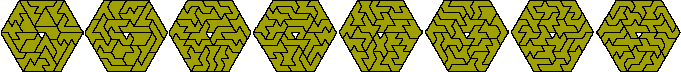Patrick has also made some other enneiamonds constructions.
Another figure which can be made with enneiamonds is this hexagon with sides 15-16-15-16-15-16.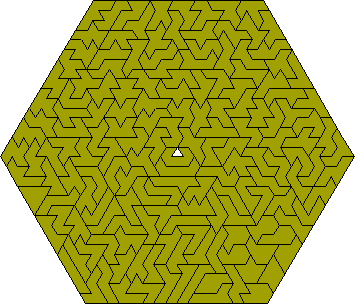There are many other possible constructions which could be made including the two triangles shown below.Since 160 = 13- 9 we could make 13-fold copies of each of the enneiamonds with 9 holes in each triangle. Two possible examples of this are shown below. With all the holes in these figures they would probably be very hard to make.After 14 hours of computer time Patrick Hamlyn has found a solution to the first one of the above.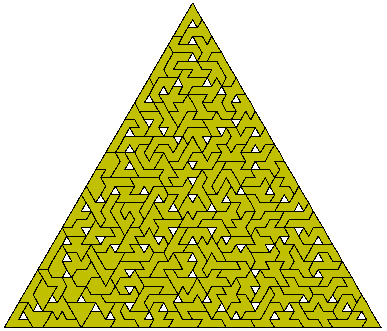Another easier possibility is the making of an 11-fold copy of a dodekiamond as in this example. The four pieces at the right can solve this problem for 1052 of the 3334 dodekiamonds. The six constructions below provide solutions to another 59 of the problems.The 159 enneiamonds without a hole cannot form a parallelogram but there are two possible trapezia shown here. Both are made up from made up of three trapezia - the first could be rearranged to form the second.. The bottom figure shows another example of three congruent shapes made with the set.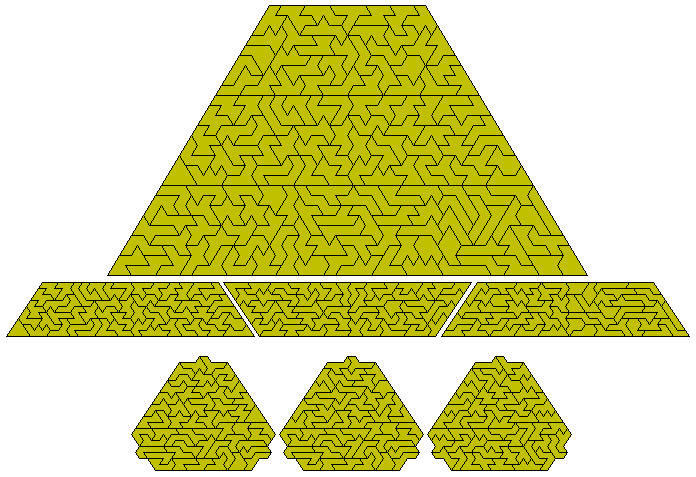Multiple enlargments of a piece can be made. These examples show sets of 7, 10,12 and 13 enlargements based on 159 = 22 + 22 + 32 + 42 + 42 + 52 + 62 + 72, 159 = 12 + 22 + 22 + 22 + 22 + 22 + 22 + 22 + 72 + 92, 159 = 22 + 22 + 22 + 22 + 22 + 22 + 22 + 22 + 22 + 5 2 + 72 + 72. and 159 = 12 +22 + 22 + 22 + 22 + 22 + 22 + 22 + 22 + 22 + 32 + 72 + 82.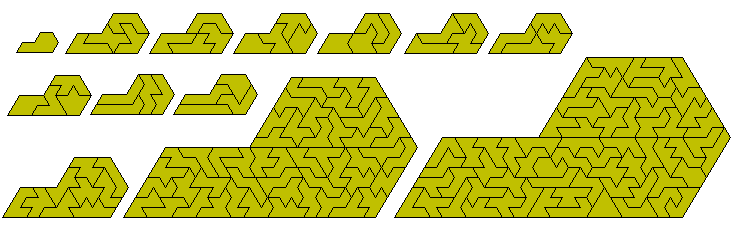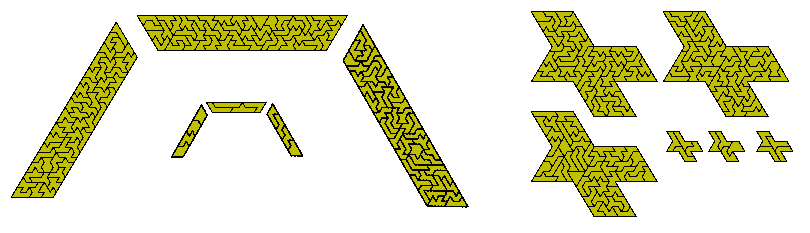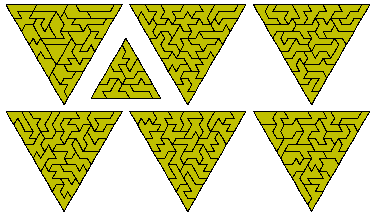The 18 piece solution below is based on 159 = 3 x (17 x 12 + 92)